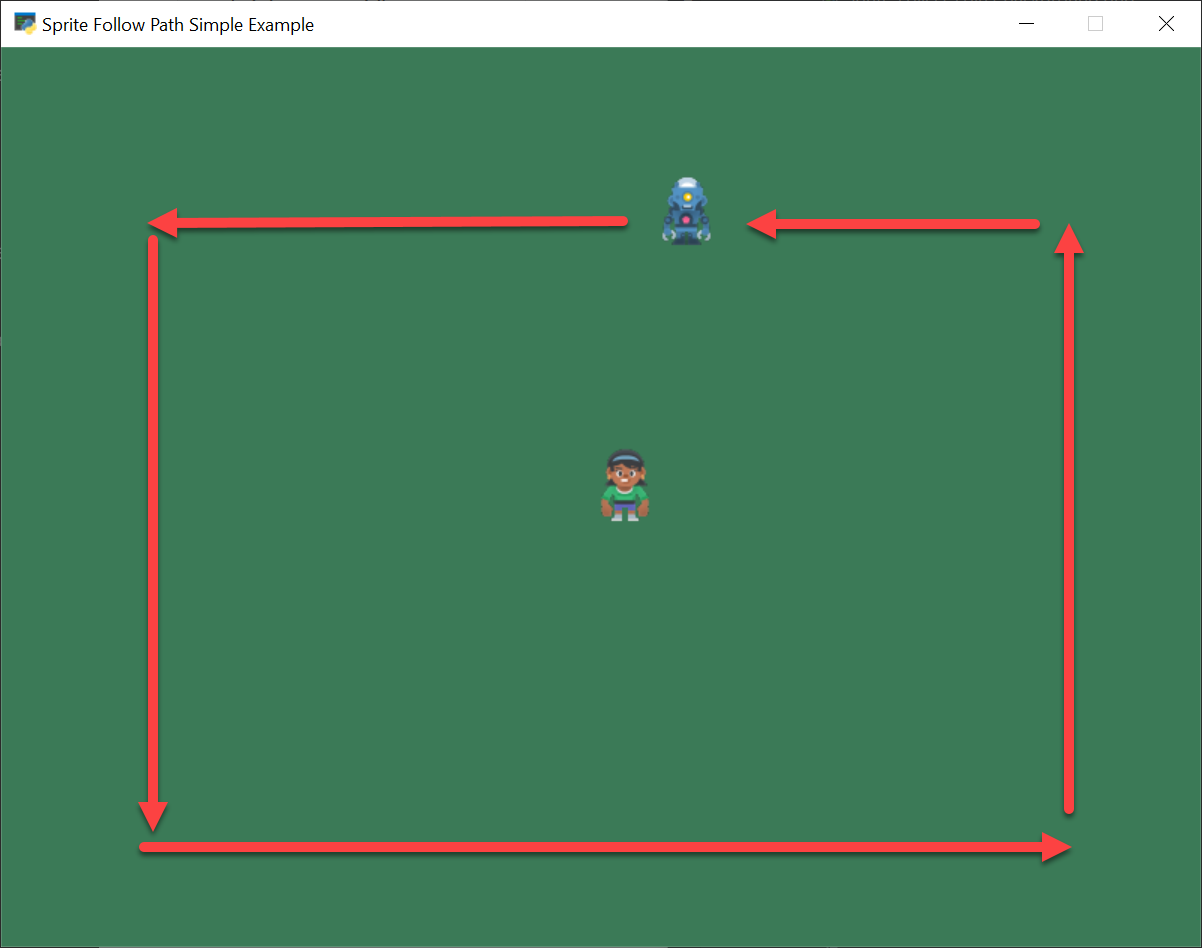# Sprites That Follow a Path#follow_path.py#
 ``` 1 2 3 4 5 6 7 8 9 10 11 12 13 14 15 16 17 18 19 20 21 22 23 24 25 26 27 28 29 30 31 32 33 34 35 36 37 38 39 40 41 42 43 44 45 46 47 48 49 50 51 52 53 54 55 56 57 58 59 60 61 62 63 64 65 66 67 68 69 70 71 72 73 74 75 76 77 78 79 80 81 82 83 84 85 86 87 88 89 90 91 92 93 94 95 96 97 98 99 100 101 102 103 104 105 106 107 108 109 110 111 112 113 114 115 116 117 118 119 120 121 122 123 124 125 126 127 128 129 130 131 132 133 134 135 136 137 138 139 140 141 142 143 144 145 146 147 148 149 150 151 152 153 154 155 156 157 158 159 160 161 162 163 164``` ```""" Sprite Follow Path This example has enemy sprites follow a set path. Artwork from https://kenney.nl If Python and Arcade are installed, this example can be run from the command line with: python -m arcade.examples.sprite_follow_path """ import arcade import math # --- Constants --- SPRITE_SCALING_PLAYER = 0.5 SPRITE_SCALING_ENEMY = 0.5 ENEMY_SPEED = 3.0 SCREEN_WIDTH = 800 SCREEN_HEIGHT = 600 SCREEN_TITLE = "Sprite Follow Path Simple Example" class Enemy(arcade.Sprite): """ This class represents the Enemy on our screen. """ def __init__(self, image, scale, position_list): super().__init__(image, scale) self.position_list = position_list self.cur_position = 0 self.speed = ENEMY_SPEED def update(self): """ Have a sprite follow a path """ # Where are we start_x = self.center_x start_y = self.center_y # Where are we going dest_x = self.position_list[self.cur_position] dest_y = self.position_list[self.cur_position] # X and Y diff between the two x_diff = dest_x - start_x y_diff = dest_y - start_y # Calculate angle to get there angle = math.atan2(y_diff, x_diff) # How far are we? distance = math.sqrt((self.center_x - dest_x) ** 2 + (self.center_y - dest_y) ** 2) # How fast should we go? If we are close to our destination, # lower our speed so we don't overshoot. speed = min(self.speed, distance) # Calculate vector to travel change_x = math.cos(angle) * speed change_y = math.sin(angle) * speed # Update our location self.center_x += change_x self.center_y += change_y # How far are we? distance = math.sqrt((self.center_x - dest_x) ** 2 + (self.center_y - dest_y) ** 2) # If we are there, head to the next point. if distance <= self.speed: self.cur_position += 1 # Reached the end of the list, start over. if self.cur_position >= len(self.position_list): self.cur_position = 0 class MyGame(arcade.Window): """ Our custom Window Class""" def __init__(self): """ Initializer """ # Call the parent class initializer super().__init__(SCREEN_WIDTH, SCREEN_HEIGHT, SCREEN_TITLE) # Variables that will hold sprite lists self.player_list = None self.enemy_list = None # Set up the player info self.player_sprite = None self.score = 0 # Don't show the mouse cursor self.set_mouse_visible(False) arcade.set_background_color(arcade.color.AMAZON) def setup(self): """ Set up the game and initialize the variables. """ # Sprite lists self.player_list = arcade.SpriteList() self.enemy_list = arcade.SpriteList() # Score self.score = 0 # Set up the player # Character image from kenney.nl self.player_sprite = arcade.Sprite(":resources:images/animated_characters/female_person/" "femalePerson_idle.png", SPRITE_SCALING_PLAYER) self.player_sprite.center_x = 50 self.player_sprite.center_y = 50 self.player_list.append(self.player_sprite) # List of points the enemy will travel too. position_list = [[50, 50], [700, 50], [700, 500], [50, 500]] # Create the enemy enemy = Enemy(":resources:images/animated_characters/robot/robot_idle.png", SPRITE_SCALING_ENEMY, position_list) # Set initial location of the enemy at the first point enemy.center_x = position_list enemy.center_x = position_list # Add the enemy to the enemy list self.enemy_list.append(enemy) def on_draw(self): """ Draw everything """ self.clear() self.enemy_list.draw() self.player_list.draw() def on_mouse_motion(self, x, y, dx, dy): """ Handle Mouse Motion """ # Move the center of the player sprite to match the mouse x, y self.player_sprite.center_x = x self.player_sprite.center_y = y def on_update(self, delta_time): """ Movement and game logic """ self.enemy_list.update() def main(): """ Main function """ window = MyGame() window.setup() arcade.run() if __name__ == "__main__": main() ```